# 1 Introduction

This tutorial is an introduction to nonlinear regression models in SAS. Some additional knowledge of SAS may be useful for understanding certain aspects of this material. For more information on these requirements, the reader is referred to the tutorials on the Data Step and Graphics and Plotting.

Nonlinear models are often associated with functions that curve or are not straight lines. This, however, is a not a precise definition. Linear or nonlinear in statistical models refers to how the model parameters enter the model function. Some models that graphically appear “curved” are, in fact, linear models. For example, a quadratic model is graphically a concave or convex shape, but the model is linear:

$y_{i} = \beta_0 + \beta_1 \cdot x_{1} + \beta_2 \cdot x_{1}^2 + \epsilon_{i}$ In this model, the parameters $$\beta_0$$, $$\beta_1$$, and $$\beta_2$$ are additive relative to one another making this a linear model. An example of a nonlinear model would be:

$y_{i} = \beta_0\cdot x_{i}^{\beta_1} + \epsilon_{i}$

where the parameters $$\beta_0$$ and $$\beta_1$$ are multiplicative relative to each other. In terms of estimation, this makes a difference. In linear models, solutions for parameter estimates can be derived algebraically, while no closed form solutions or equations can be derived for nonlinear models. Estimation in nonlinear models is obtained through an iterative “trial-and-error” process, the details of which can vary and are only covered briefly here. For more technical material on nonlinear estimation methods, see texts such as Nonlinear Regression Analysis and Its Applications.

Regardless of the methodology, the iterative estimation process typically requires the user to specify starting values for each parameter. These are basically guesses at what the parameters might be to start the process. The user may have some clues from the data, or they may be completely unknown to the user. Such details will depend on the model used, knowledge of the process being modeled, and the data itself. Examples for these aspects will be demonstrated below.

##### 1.0.0.0.1 Note: Linearization

The ability to estimate nonlinear models is, computationally, relatively recent. Before this ability was available, nonlinear model forms were often transformed or linearized such that they became linear with additive parameters. This made estimation through standard linear regression possible. An example would be the power model above:

$y_{i} = \beta_0\cdot x_{i}^{\beta_1} + \epsilon_{i}$

By taking the logarithm of the model, we get a linear form:

$ln(y_{i}) = ln(\beta_0) + \beta_1\cdot x_{i}^{} + \phi_{i}$ $y_{i}^*= \beta_0^* + \beta_1\cdot x_{i} + \phi_{i}$ where $$y_{i}^*$$ is the logarithm of the response, $$y_{i}$$, and the intercept of this model, $$\beta_0^*$$, is the logarithm of the original parameter $$\beta_0$$. The exponential function could then be used to untransform the predicted values and intercept parameter. Note, however, that the error term $$\phi_{i}$$ is not equivalent to the additive error $$\epsilon_{i}$$. Thus, linearization provides only an approximation to the desired nonlinear form. For these reasons, it is now more desirable to use true nonlinear estimation techniques rather than linearization. In some cases, however, linearization may be useful for deriving the starting values necessary for the nonlinear estimation process.

# 2 Model Selection

There are an infinite number of nonlinear forms that can be selected for representing data. The selection process, however, should follow some basic guidelines. First and foremost, model selection, and the use of nonlinear modeling in general, should meet the research objectives. What is the purpose of modeling and what does the researcher hope to gain from it? A second and related factor is model interpretation. Are the parameters of the model interpretable in biological terms? Do they have biological relevance? This is often determined from theoretical considerations or previous research. Given these decisions, the data itself are an important consideration. What pattern does the data generally have? Is it increasing, decreasing, curved, etc.? Are there sufficient data points to support estimation of a model and do they fall in appropriate places to provide good estimation? As an example, many models attempt to estimate minimum or maximum theoretical values (asymptotes) when the values of dose or time are very large. Even though such doses or times are not practically relevant, they are important for model estimation. If the data set lacks points at large dose/time values, then the estimations will be poor, or even fail. Lastly, the complexity of model forms and their parameterization should be considered. Models containing many parameters (often more than 3) or parameters that involve exponents, ratios, or other multiplicative relationships can be difficult to estimate. Models should be as concise as possible with the fewest parameters as possible (parsimony). In many cases, parsimony and complexity issues can be resolved by reparameterizing the model or selecting a different model to make a simpler form for estimation.

# 3 Common Nonlinear Models

## 3.1 Exponential Forms

Exponential forms are often used to describe uniformly decreasing or increasing curved trends found in response decay or growth. They are typically two to three parameter models. Two common forms used in agricultural research are:

$y = a*exp(-b*x) + c \tag*{(Decreasing)}$ $y = M - a*exp(-b*x) \tag*{(Increasing)}$ In these models, the parameter $$a$$ is releated to an intercept at $$x=0$$, $$b$$ is a rate related parameter, $$M$$ is an upper asymptote, and $$c$$ is a lower asymptote, both approached as $$x$$ grows larger. In many cases, $$c$$ is often assumed to be zero and $$M$$ may be set to 1.0 or 100 for proportional or percentage data, respectively.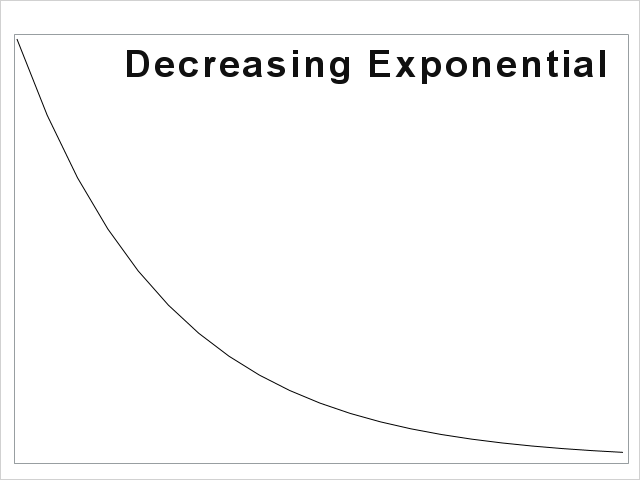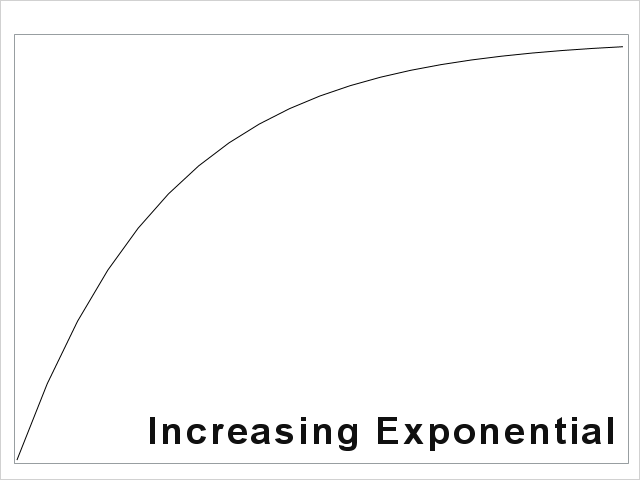## 3.2 S-Curve Forms

Similar to the exponential forms, S-curves may be either increasing or decreasing. A key difference, however, is that these forms have an inflection point midway where the rate of change in the curve slows and changes direction giving the curves their characteristic “S” shape. They are commonly used to describe dose-response relationships or growth over time. Many are taken directly from the functional forms of cumulative probability distributions for theoretical reasons (for example probit, tobit and logit models). Others are derived from mathematical derivations of growth or decay. Some specific types of S-curves are outlined below.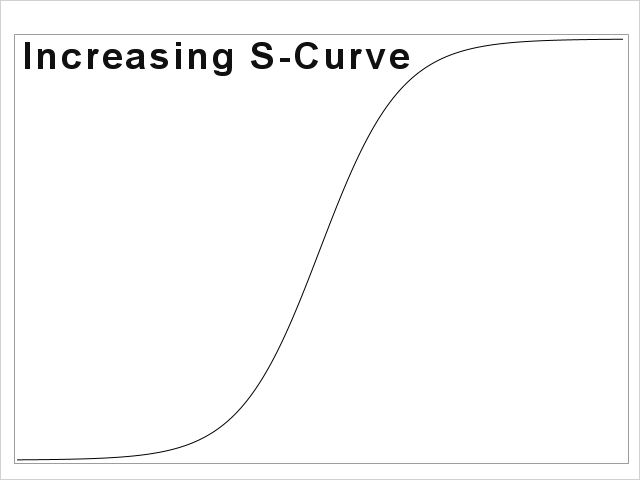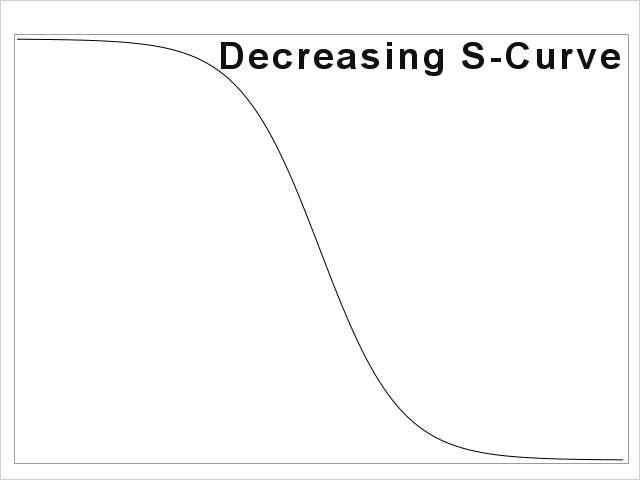### 3.2.1 Logistic Curve

The logistic curve (not to be confused with logistic regression) is often seen in dose-response work with a probabilistic interpretation, or from differential equations describing growth. Its parameterization can vary widely. The form below is often seen in agricultural dose-response work.

$y = \frac{M}{1 + exp(K*(x - L))}$ Here, $$M$$ is an upper asymptote representing a theoretical maximum of the response, $$y$$. $$K$$ is a rate parameter. When $$K$$ is positive, the curve begins close to $$M$$ and decreases. When negative, the curve starts close to zero and increases to $$M$$. The parameter $$L$$ is, by default, the infection point at 50% of $$M$$. That is, when the variable $$x$$ equals $$L$$ the response $$y$$ equals $$\frac{M}{2}$$. In dose-response work, this 50th percentile is commonly known as the $$LD_{50}$$ or $$ED_{50}$$. In growth models, $$L$$ represents a quantification of lag time for the start of growth. The quantity $$(x - L)$$ often appears in logrithmic form as this transformation makes the data pattern symmetric about the inflection point (as in the data examples below). Hence, the form may appear as $$(ln(x) - L)$$, $$ln(\frac{x}{L})$$, or an equivalent. It is also possible to have $$L$$ estimate other percentiles by reparameterizing the function (see for example Price, et. al, 2017.

### 3.2.2 Probability Based Models

These encompass a wide variety of models that were traditionally used for dose-response modeling. Examples include cumulative distributions for the Normal (probit), t (tobit), and logistic (logit). Because these functions are complex and involve integration to compute, they are not readily written out in equation form, but instead either use predefined probability functions in SAS, or dedicated SAS procedures (Proc Probit).

### 3.2.3 Asymmetric S-Curves

All the curves described above exhibit symmetry about the inflection point. As mentioned, sometimes asymmetry in the data is accommodated through logrithmic transformations in the model. There are some models, however, that can directly assume asymmetry. Two examples of this are the Weibull and Gompherz.

$y = M*(1-exp(-((K*x)^C)))\tag*{Weibull}$

$y = = M*exp(−exp(−K*(x − L)))\tag*{Gomphertz}$ In these forms, $$M$$, $$K$$, and $$L$$ play similar roles as those in the logistic model above, although $$L$$ does not relate to the 50th percentile.

### 3.2.4 Hormesis Models

There are a special class of dose-response models where the data generally follow the decreasing S-Curve shape, but they also exhibit a small initial increase at very low doses. This behavior, for example, is often observed in some herbicides that are similar to growth hormones and small doses actually induce growth, rather than plant injury. This process is referred to as hormesis and one model to describe this phenomenon was given by Brain and Cousens, 1989:

$y = \frac{(M + f*x)}{1 + exp(B*(log(x)-log(L)))}$ This model is similar to the logistic model above with the addition of the parameter $$f$$ which creates the bump in the curve at small doses, $$x$$. An example of fitting this curve can be found at Price, et. al, 2017

# 4 Model Estimation

Nonlinear estimation is an iterative process. That is, there are no closed form equations to compute parameter values. Because of this, the computer algorithms that estimate parameters will use a trial and error process to judge whether the estimates it has are “good enough”, which usually means they have met some predetermined numeric condition (referred to as convergence). This requires that the algorithm has a place to start, or starting parameter values, to begin the estimation. The algorithm will then adjust the starting values to improve to model fit and continue doing so until the model converges. Conceptually, this process is analogous to letting a marble drop into a bowl from the edge. The marble will oscillate back and forth and around until it settles at the bottom or a low spot.

Traditionally, the trial and error iterative process has been based on the concept of least squares where the objective is to find parameters such that the distance between the predicted curve and the observed points is minimal. In SAS, the least squares estimation process is carried out through the procedure Proc Nlin. While Proc Nlin is still useful, it does have limitations because, for statistical inference, it assumes the data are normally distributed in addition to assuming that all parameters in the model are fixed effects. Its benefits, however, include ease of use and good convergence on parameter solutions, which can be useful for deriving starting values to use in more sophisticated nonlinear modeling procedures.

A more modern alternative to least squares estimation is the process of maximum likelihood estimation. In maximum likelihood, the objective of the parameter iterations and adjustments is to maximize the likelihood or probability of observing the data at hand. This has the obvious advantage that the likelihood function does not have to be a normal distribution, but could, alternatively, come from a wide array of potential distributions (generalized nonlinear models). The procedure available in SAS, Proc Nlmixed, also has the ability to consider parameters as random effects, thereby giving these models more flexibility to accurately represent the data. The drawbacks here are a more sensitive and complex estimation algorithm that can show difficulty in converging on a solution. Obtaining good starting values close to the final estimates can be essential when using Proc Nlmixed. The least squares procedure, Proc Nlin above, can be a good way of deriving these.

Both least squares Proc Nlin and maximum likelihood Proc Nlmixed will be demonstrated below.

# 5 Data used in examples

## 5.1 Exponential Data

These data are a simple simulated set of variables for demonstrating the exponential model and basic nonlinear estimation and results.


data exp;
do x = 0 to 20;
do rep = 1 to 4;
y = 100*exp(-.2*x) + rannor(0934)*5;
output;
end;
end;
;

proc sgplot;
scatter x=x y=y/filledoutlinedmarkers markeroutlineattrs=(color=black) markerattrs=(symbol=circlefilled size=12) MARKERFILLATTRS=(color=green);
xaxis LABELATTRS=( Family=Arial Size=15 Weight=Bold) VALUEATTRS=(Family=Arial Size=12 Weight=Bold);
yaxis LABELATTRS=( Family=Arial Size=15 Weight=Bold) VALUEATTRS=(Family=Arial Size=12 Weight=Bold);
run;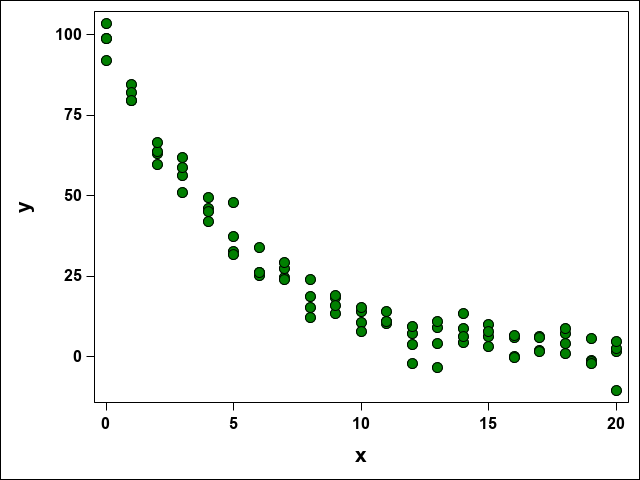## 5.2 Onion Data

These data are from an experiment assessing the germination of onion seeds under varying osmotic pressure (available moisture). In the experiemnt, there were two petri dishes of 100 seeds each. This was replicated four times. The cumulative number of seeds germinated (emerged radical of 1 mm) were recorded for 20 days.


PROC IMPORT OUT= WORK.onion
DATAFILE= "./data/onion.xlsx"
DBMS=XLSX REPLACE;
sheet="Onion";
RUN;

proc sort data=onion;
by trt day;
run;

proc sgplot data=onion;
styleattrs datacolors=(cxFD07FA cx0752FD);
scatter x=day y=germ/group=trt filledoutlinedmarkers markeroutlineattrs=(color=black) markerattrs=(symbol=circlefilled size=12);;
xaxis type=log logbase=e logstyle=linear label='Day' LABELATTRS=( Family=Arial Size=15 Weight=Bold) VALUEATTRS=(Family=Arial Size=12 Weight=Bold);
yaxis label='Germination (%)' LABELATTRS=( Family=Arial Size=15 Weight=Bold) VALUEATTRS=(Family=Arial Size=12 Weight=Bold);
keylegend /AUTOITEMSIZE valueattrs=(size=14) TITLEATTRS=(weight=bold size=12) Title = 'Osmotic Pressure (Pa)';
run;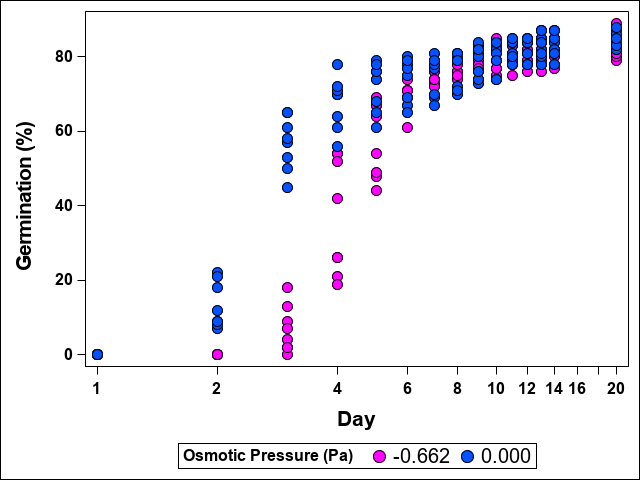## 5.3 ALS Activity in Winter Wheat

These data record the activity of Acetolactate Synthase (ALS) as % absorbtion in winter wheat under increasing doses of a herbicide that inhibits ALS (Rainbolt, C. R., et al., 2005. The study was carried out over eight replications (plates).


PROC IMPORT OUT= WORK.ALS
DATAFILE= "./data/ALS.xlsx"
DBMS=XLSX REPLACE;
sheet="ALS";
RUN;

proc sgplot;
scatter x=dose y=percent/FILLEDOUTLINEDMARKERS markerattrs=(symbol=circlefilled size=14) MARKERFILLATTRS=(color=tan) MARKEROUTLINEATTRS=(color=black);;
xaxis type=log logbase=e logstyle=linear label='ALS Herbicide Dose (ug/ml)' LABELATTRS=( Family=Arial Size=15 Weight=Bold) VALUEATTRS=(Family=Arial Size=12 Weight=Bold);
yaxis label="ALS Activity (% Absorbance)" LABELATTRS=( Family=Arial Size=15 Weight=Bold) VALUEATTRS=(Family=Arial Size=12 Weight=Bold);
run;